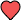# Resources

## How to Program the Quadratic Formula on Your TI 84 Graphing Calculator

Have you ever been in math class and hoped your graphing calculator could solve the problem for you? Well, there is a way at least for quadratic formula math problems by creating a simple program on your TI-84 graphing calculator.

### Steps To Program Distance Formula

1. Press prgm and slide over to NEW
2. Spell out QUAD to name the program
3. Type the following into the QUAD program:
a+bi
Prompt A,B,C
(-B−√(B²-4AC))/(2A)→D
(−B+√(B²-4AC))/(2A)→E
Disp D,E

Note: The '-' in front of both B's is a negative sign.

1. To run the program, press prgm and then search for the program QUAD© 2019 Infinity Calcs | Made with